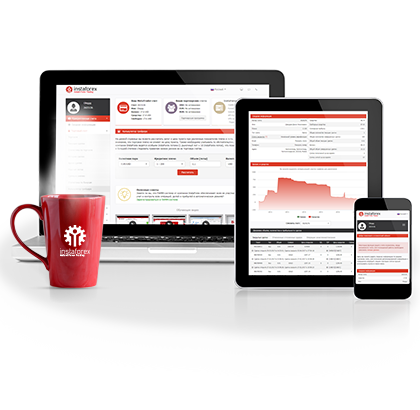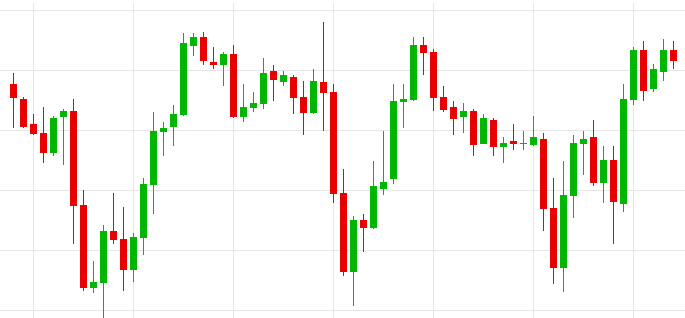Rapida apertura di un conto

Ricevere la lettera formativaPer dispositivi mobili

Bonus InstaForex
Ricaricare il conto
Prelevare fondi

Volatility: what is it and how is it calculated?

Professional traders see this term as the movement of the foreign exchange market.

The volatility of the forex market is a statistical indicator that reflects variations of prices during a certain time period.

Volatility of prices for financial instruments is an important criterion for traders. Before choosing a financial instrument, a trader needs to know what fluctuations to expect, since this determines a potential profit.It is obvious that sharp swings in a value of a particular currency increase the probability of profit. At the same time, risks of losses in case of an incorrect forecast also increase.

As a rule, currency volatility is evaluated on the basis of historical data. In other words, experts analyze a price dynamic in the past to prepare forecasts about potential volatility. This approach is quite rational since even novice market participants know the main market law saying that history is cyclical. This law allows economists to make predictions for the future, relying on price behavior in the past.

Importantly, price fluctuations do not occur on their own. Therefore, it is essential, to understand what determines volatility of a currency. The strongest movements are often triggered by fundamental factors that can affect market volatility, specifically, publication of important economic reports, monetary policy decisions made by the global central banks, and other events in the political sphere of a country. However, currency volatility may increase amid speculative or psychological reasons as the market responds to anything.

Calculation of volatility

The arsenal of a forex trader includes many tools that can considerably facilitate the trading process, making complex calculations redundant. Today, market volatility can be measured on the basis of volatility charts. However, price volatility can be determined both visually and through formulas.

There are several formulas for calculating price volatility that take into account peculiarities of a particular financial asset.

Formula for calculating currency volatilitywhere,is a standard deviation of an asset's profitability and P is a time period in annual terms.

The average values ofare known for every financial instrument. Let us suppose that the deviation equals 0.01 per one trading day.

Importantly, calculations are done for the average annual volatility. As there are 252 trading days in a year and as we applyto one trading day, then the time period P is equal to 1/252.

After filling the obtained values in the formula, we received the following result:As you see, it is quite easy.

If you need to get the indicator of volatility for a certain period of time, then the formula takes the following form:Whereis the average annual volatility and T is a given period of time.

Let us suppose that T equals one month or 1/12 of a year. In this case the calculation looks like this:Any trader or investor can calculate the historic volatility.

To develop a trading strategy, a forex trader chooses a method of analysis and creates a risk management system through personal preferences. Besides, a trader is strongly recommended to select carefully a trading instrument to work with in accordance with the volatility factor. A correct estimate of price volatility is one of the key components of success in the market.